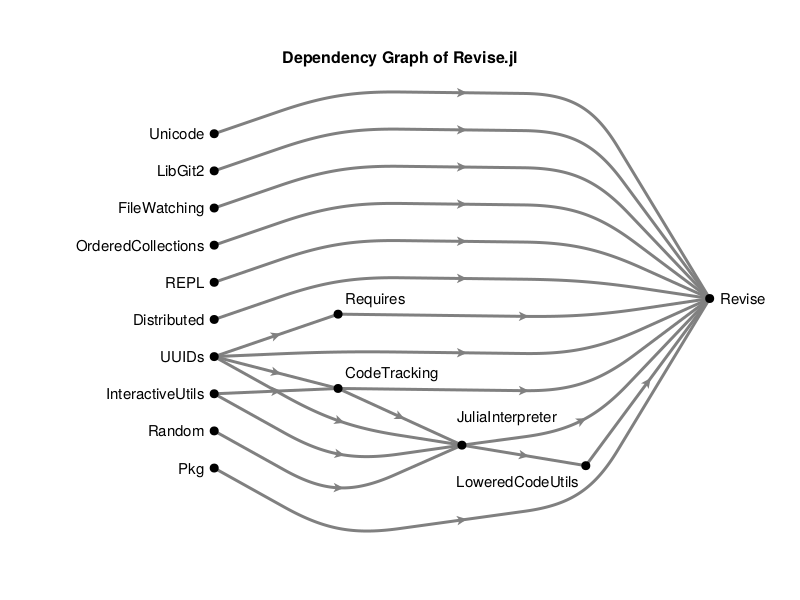# Dependency Graph of a Package

In this example we'll plot a dependency graph of a package using PkgDeps.jl and and a DAG layout from LayeredLayouts.jl

using CairoMakie
using GraphMakie
using Graphs
using LayeredLayouts
using PkgDeps
using Makie.GeometryBasics
using Makie.Colors

First we need a small function which goes recursively through the dependencies of a package and builds a SimpleDiGraph object.

function depgraph(root)
packages = [root]
connections = Vector{Pair{Int,Int}}()

for pkg in packages
pkgidx = findfirst(isequal(pkg), packages)
deps = direct_dependencies(pkg)

for dep in keys(deps)
idx = findfirst(isequal(dep), packages)
if idx === nothing
push!(packages, dep)
idx = lastindex(packages)
end
push!(connections, idx => pkgidx)
end
end
g = SimpleDiGraph(length(packages))
for c in connections
end
return (packages, g)
end

As an example we'll plot the dependency Graph of Revise.jl because it is one of the most important packages in the Julia ecosystem but does not have a huge dependency tree.

(packages, g) = depgraph("Revise")
N = length(packages)
xs, ys, paths = solve_positions(Zarate(), g)

# we scale the y coordinates so the plot looks nice in DataAspect()
ys .= 0.3 .* ys
foreach(v -> v .= 0.3 .* v, values(paths))

In GraphMakie the layout always needs to be function. So we're creating a dummy function... We will use the Edge waypoints attribute to get the graph with the least crossings.

lay = _ -> Point.(zip(xs,ys))
# create a vector of Point2f per edge
wp = [Point2f.(zip(paths[e]...)) for e in edges(g)]

# manually tweak some of the lable aligns
align = [(:right, :center) for i in 1:N]
align  = (:left, :center)  # Revise
align  = (:right, :top)    # LoweredCodeUtils
align  = (:left, :bottom)  # CodeTracking
align = (:left, :bottom)  # JuliaInterpreter
align = (:left, :bottom)  # Requires

# shift "JuliaInterpreter" node in data space
offset = [Point2f(0,0) for i in 1:N]
offset = Point(-0.1, 0.1)

f, ax, p = graphplot(g; layout=lay,
arrow_size=15,
edge_color=:gray,
nlabels=packages,
nlabels_align=align,
nlabels_distance=10,
nlabels_textsize=15,
nlabels_offset=offset,
node_size=[9.0 for i in 1:N],
edge_width=[3 for i in 1:ne(g)],
waypoints=wp,
ax.title = "Dependency Graph of Revise.jl"
xlims!(ax, -0.6, 5.6)
hidedecorations!(ax); hidespines!(ax); ax.aspect = DataAspect()If you run this example using GLMakie you can add this code to play around with the interactive features.

deregister_interaction!(ax, :rectanglezoom)
register_interaction!(ax, :nodehover, NodeHoverHighlight(p))
register_interaction!(ax, :edgehover, EdgeHoverHighlight(p))
register_interaction!(ax, :edrag, EdgeDrag(p))
register_interaction!(ax, :ndrag, NodeDrag(p))